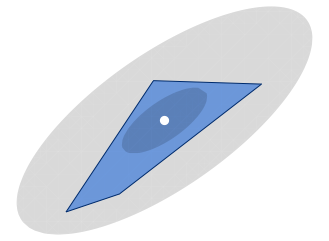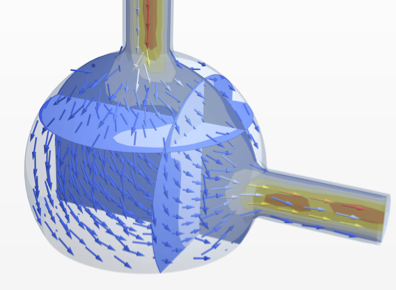# Symbolic & Numeric Computation

## Asymptotics

Asymptotic methods represent a third mode of computing that complements exact symbolic and approximate numeric modes of computing for calculus and algebra. Asymptotic methods are what disciplines turn to when they run into hard problems and are used in a wide variety of areas, including number theory, analysis of algorithms, statistics, theoretical physics and numerical methods. Asymptotic computing allows hard problems to be solved and furthermore is typically more insightful even when other modes of computing succeed. With Version 12, asymptotic computing is fully automated and as easy to use as other high-level solvers.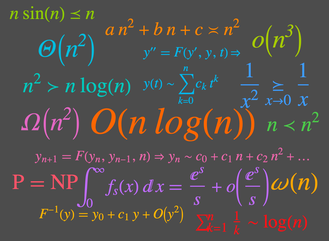## Core Calculus

Calculus may seem by now a completely mature topic, but nothing could be further from the truth. Calculus is growing in the number of people that study it across the world, it is being absorbed at an ever younger age, and it is being used because of the power of models across an ever-expanding range of disciplines. The calculus functionality in the Wolfram Language, already the the strongest in the world, improves dramatically in Version 12. New calculus topics are automated, existing functions are strengthened and the whole subsystem is re-engineered for learnability and applicability.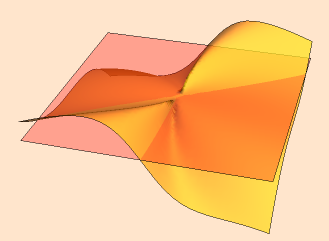## Algebraic Computation

Version 12 of the Wolfram Language extends its already strong capabilities in algebraic computation with functionality for users ranging from pre-college students to researchers. Improvements span from new functions that manipulate equations step by step to more powerful equation and optimization solvers to equational theorem proving.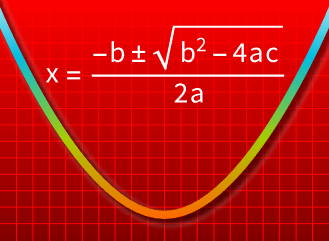## Convex Optimization

Version 12 expands the scope of optimization solvers in the Wolfram Language to include optimization of convex functions over convex constraints. Convex optimization is a class of problems for which there are fast and robust optimization algorithms, both in theory and in practice. Just as advances in linear optimization opened up many industrial applications, ever-wider classes of problems are being identified to be convex in a wide variety of domains, such as statistics, finance, signal processing, geometry and many more.